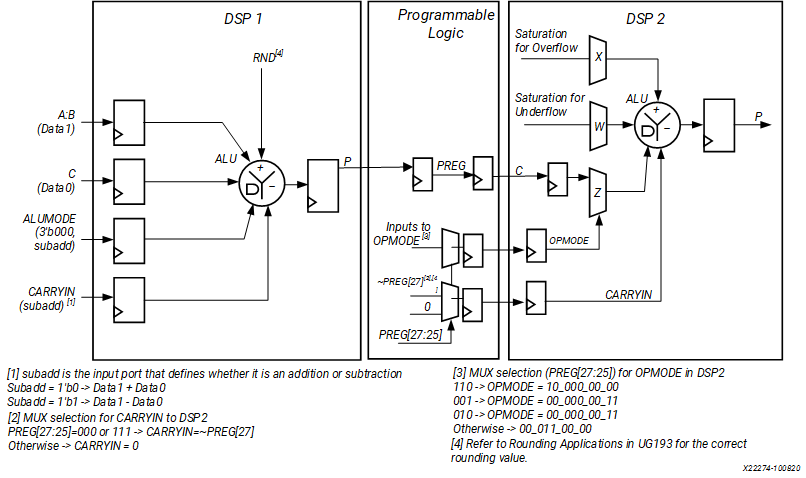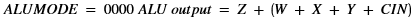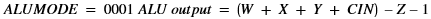# Rounding and Saturation for Adder

## Versal ACAP DSP Engine Architecture Manual (AM004)

Document ID
AM004
Release Date
2022-09-11
Revision
1.2.1 English

A saturate (SAT) and round (RND) function for addition/subtraction operation can be provided by adding a second DSP58 and by using some P output bits from the previous DSP58 to detect the SAT condition. The following figure shows a simplified block diagram of the implementation.

Figure 1. Rounding and Saturation for AdderProgrammable logic is used to implement multiplexers controlled by the P output bits of the first DSP to generate OPMODE and CARRYIN for the second DSP58. The operands for the addition/subtraction are fed to the first DSP58 through the concatenation of A:B and C. The rounding value is applied to the WMUX (static rounding to infinity is implemented). In the use case design, there is a dedicated input pin (subadd) that decides whether the inputs are added or subtracted. Considering that the output is represented as 26 bits (8 bits of the integer part and 18 bits of the fractional part), the saturation conditions (positive or negative magnitude) are detected by checking bits 27, 26, and 25 of P output from the first DSP. Bit 25 is the sign bit. Bits 26 and 27 are guard bits. The second guard bit is needed because in the worst case extra overflow due to the RND addition can occur. In the case of saturation, a unique value at the input of the X and W MUXes is selected respectively as ALU output. The output (8 bits of integer part) will be in a decimal representation of +127 for the positive range and –128 for the negative range.

Operations on W, X, Y, Z, and CIN are controlled by setting ALUMODE as follows: